Name:    Chapter 9 Pre Test

Multiple Choice
Identify the choice that best completes the statement or answers the question.

1.

Write the first five terms of the sequence. (Assume that n begins with 1.)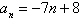a. 1, –14, –21, –28, –35 b. 1, –6, –13, –20, –27 c. 1, 9, 17, 25, 33 d. 8, 1, –6, –13, –20 e. –15, –22, –36, –36, –43

2.

Find the sum.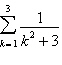a.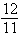b.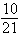c.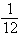d. 1 e.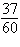3.

Determine whether the sequence is arithmetic. If so, find the common difference.
8, 13, 18, 23, 28
 a. –5 b. 3 c. not arithmetic d. 8 e. 5

4.

Determine whether the sequence is arithmetic. If so, find the common difference. (Assume that n begins with 1.)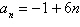a. 1 b. –1 c. 6 d. not arithmetic e. –6

5.

Find a formula for an for the arithmetic sequence.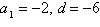a.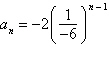b.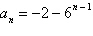c.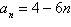d.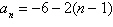e.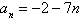6.

Write the first five terms of the arithmetic sequence.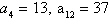a. 7, 10, 13, 16, 19 b. 4, 7, 11, 15, 19 c. 4, 1, –2, –5, –8 d. 4, 12, 36, 108, 324 e. 4, 7, 10, 13, 16

7.

Write the nth term of the arithmetic sequence as a function of n.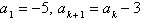a.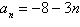b.c.d.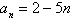e.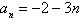8.

Find the indicated nth partial sum of the arithmetic sequence.
1.9, 4.8, 7.7, 10.6, ...., n=20
 a. 588 b. 590 c. 589 d. 419 e. 647

9.

Write the first five terms of the geometric sequence.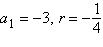a.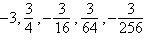b.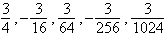c. –3, 12, –48, 192, –768 d. –3, –7, –11, –15, –19 e.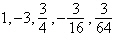10.

Write the nth term of the geometric sequence as a function of n.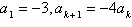a.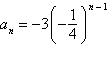b.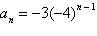c.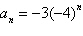d.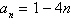e.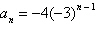11.

Match the geometric sequence with its graph from the choices below.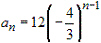a.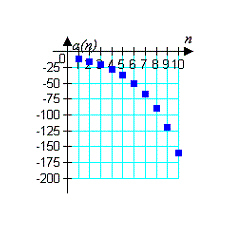d.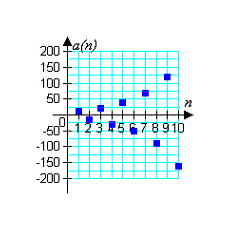b.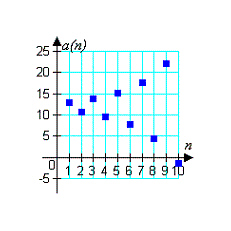e.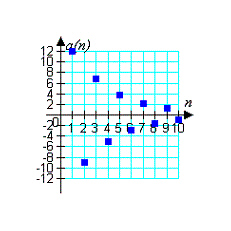c.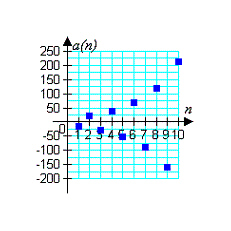12.

Find the sum using the formulas for the sums of powers of integers.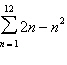a. –1482 b. –494 c. –374 d. –120 e. 78

13.

Find a quadratic model for the sequence with the indicated terms.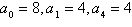a.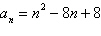b.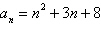c.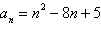d.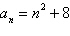e.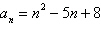14.

Use the Binomial Theorem to expand and simplify the expression.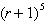a.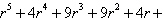b.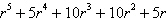c.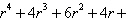d.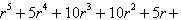e.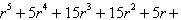15.

Use the Binomial Theorem to expand the complex number. Simplify your result.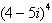a.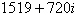b.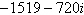c.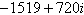d. 256 e.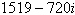16.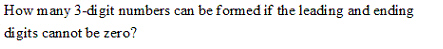a.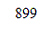b.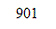c.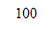d.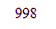e.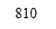17.

Nine weightlifters are competing in the dead-lift competition. In how many ways can the weightlifters finish first, second, and third (no ties)?
 a. 3 b. 27 c. 729 d. 504 e. 6

18.

Find the number of diagonals of a nonagon (9 sides). (A line segment connecting any two nonadjacent vertices is called a diagonal of the polygon.)
 a. 9 b. 27 c. 81 d. 18 e. 72

19.

Solve for n.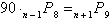a.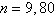b. no solution c.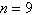d.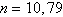e.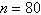20.

You are given the probability that an event will not happen. Find the probability that the event will happen.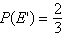a.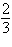b. 1 c.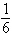d.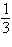e. 0